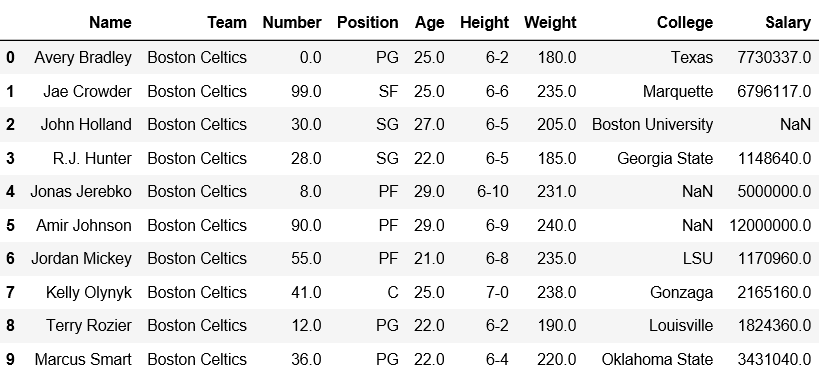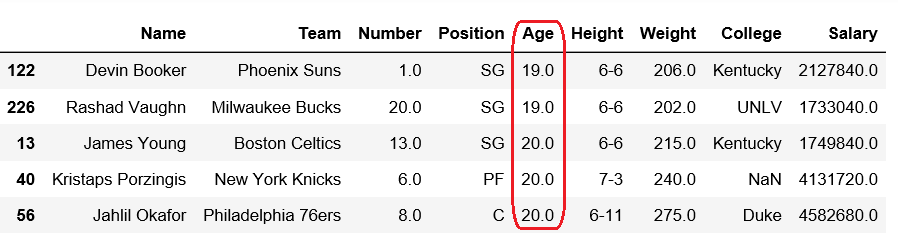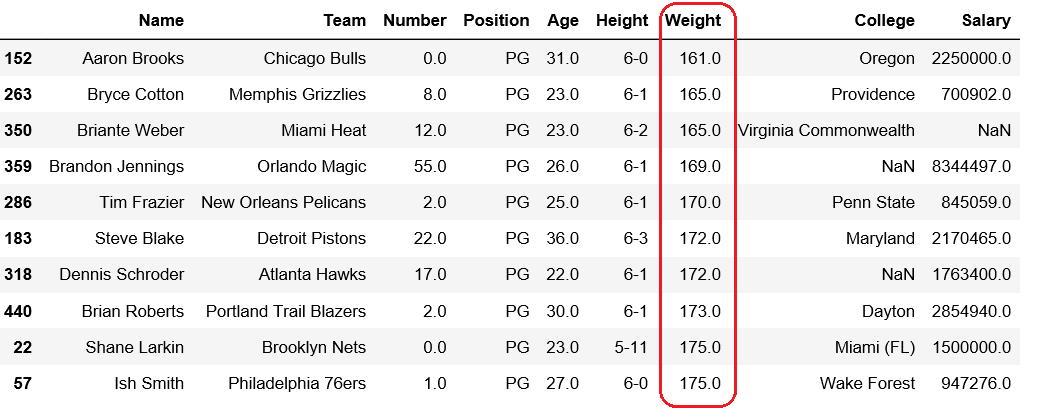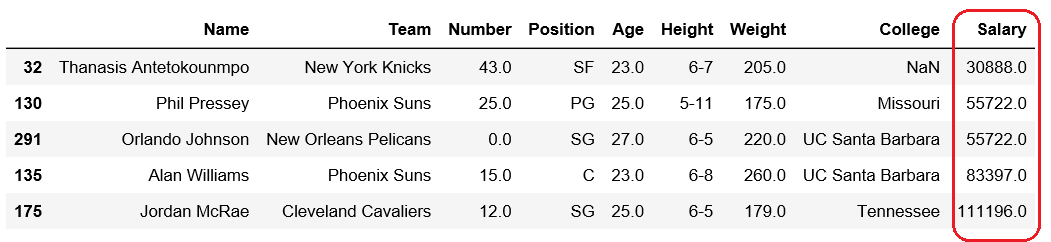Open In App
Related Articles
• Write an Interview Experience
• Pandas Exercises and Programs
• Different ways to create Pandas Dataframe

# Get n-smallest values from a particular column in Pandas DataFrame

Pandas DataFrame is two-dimensional size-mutable, potentially heterogeneous tabular data structure with labeled axes (rows and columns).

Let’s see how can we can get n-smallest values from a particular column in Pandas DataFrame.

Observe this dataset first. We’ll use ‘Age’, ‘Weight’ and ‘Salary’ columns of this data in order to get n-smallest values from a particular column in Pandas DataFrame.

 `# importing pandas module ``import` `pandas as pd ``   ` `# making data frame ``df ``=` `pd.read_csv(``"https://media.geeksforgeeks.org/wp-content/uploads/nba.csv"``) `` ` `df.head(``10``)`Code #1: Getting 5 smallest Age

 `# importing pandas module ``import` `pandas as pd ``   ` `# making data frame ``df ``=` `pd.read_csv(``"nba.csv"``) `` ` ` ` `df.nsmallest(``5``, [``'Age'``])`

Output:Code #2: Getting 10 minimum weights

 `# importing pandas module ``import` `pandas as pd ``   ` `# making data frame ``df ``=` `pd.read_csv(``"nba.csv"``) `` ` ` ` `df.nsmallest(``10``, [``'Weight'``])`

Output:Code #3: Getting 10 minimum salary

 `# importing pandas module ``import` `pandas as pd ``   ` `# making data frame ``df ``=` `pd.read_csv(``"nba.csv"``) `` ` ` ` `df.nsmallest(``5``, [``'Salary'``])`

Output: